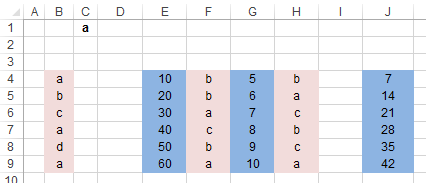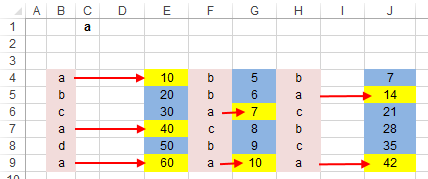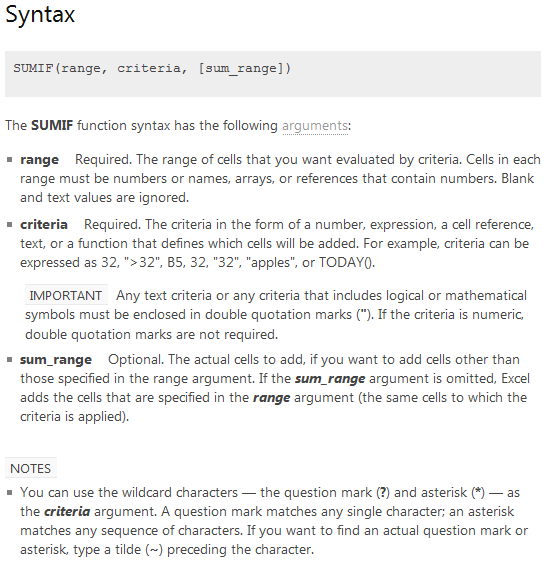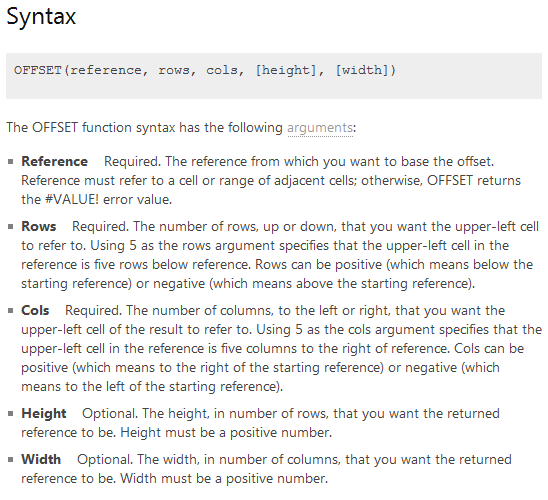# Formula Forensics No. 029 SumIf with Inconsistent Column Layouts

About a month ago, Fred, asked a question at the Chandoo.org Forums:

“I have 2 formulae one using sumif and the other one using sumproduct. Both get the same correct answer but they look a bit too long to me and I can’t find a shorter way to express.

basically I need to find out the sales volume by names and I have names on columns W, AA and AC. There are sales figures on columns Z, AB and AD that goes respectively to the name columns.

Here are my formulae. X1 is the name I’d type in to find out the combined sales figures

vs.

or do any of you have an even better idea? Any suggestion?”

Haseeb A followed up with a neat solution that used both the Sumproduct() and Sumif() functions and threw in two Offset() functions just for fun, namely:

=SUMPRODUCT(SUMIF(OFFSET(B4:B9,,{0,4,6}),C1,OFFSET(E4:E9,,{0,2,5})))

Note: the Column Numbers have been altered from the original post

So today at Formula Forensics we will pull apart Haseeb A’s formula and see what makes it tick.

## The Problem

First lets look at the data and describe Fred’s problem

Fred wants to add up the values in Columns E, G & J when the preceding Columns B, F & H contain a value which is in cell C1 or “a”This is shown diagrammatically below:The solution is 183 = 10+40+60+7+10+14+42

Fred had a Sumproduct based solution:

=SUMPRODUCT((\$B\$4:\$B\$9=\$C\$1)*(\$E\$4:\$E\$9)) + SUMPRODUCT((\$F\$4:\$F\$9=\$C\$1)*(\$G\$4:\$G\$9)) + SUMPRODUCT((\$H\$4:\$H\$9=\$C\$1)*(\$J\$4:\$J\$9))

Which is simply 3 Sumproduct formulas, one for each column pair, with separate criteria in each

Fred also had a Sumif based solution:

=SUMIF(B4:B9,C1,E4:E9)+SUMIF(F4:F9,C1,G4:G9)+SUMIF(H4:H9,C1,J4:J9)

Which similarly is 3 Sumif formulas, one for each column pair, with separate criteria in each

Haseeb A’s solution: =SUMPRODUCT(SUMIF(OFFSET(B4:B9,,{0,4,6}),C1,OFFSET(E4:E9,,{0,2,5})))

Combines a Sumproduct(), Sumif() and two Offset() functions to do the same as the above two formulas.

It is actually 2 characters longer than Fred’s Sumif’s based formula but this is quickly overcome if further ranges are added.

## Hasseb A’s Solution

Haseeb A’s formula

=SUMPRODUCT(SUMIF(OFFSET(B4:B9,,{0,4,6}),C1,OFFSET(E4:E9,,{0,2,5})))

Is based around the Excel Sumproduct() function.

=SUMPRODUCT(SUMIF(OFFSET(B4:B9,,{0,4,6}),C1,OFFSET(E4:E9,,{0,2,5}) ) )

As we saw in Formula Forensics 007, Sumproduct, Sums the Products of the included arrays.

In this case there is only a single included array consisting of a Sumif() function and so Sumproduct will simply Sum the values returned from the Sumif() function.

### Lets look at the Sumif() function.

SUMIF(OFFSET(B4:B9,,{0,4,6}),C1,OFFSET(E4:E9,,{0,2,5}))

The Excel Sumif() function has the following syntax:In our example: SUMIF(OFFSET(B4:B9,,{0,4,6}), C1, OFFSET(E4:E9,,{0,2,5}))

Range: OFFSET(B4:B9,,{0,4,6})

Criteria: C1

Sum_Range: OFFSET(E4:E9,,{0,2,5})

This reads as follows: Sum the Range OFFSET(E4:E9,,{0,2,5}) when the Range OFFSET(B4:B9,,{0,4,6}) is equal to the value in cell C1.

What are these OFFSET(B4:B9,,{0,4,6}) and OFFSET(E4:E9,,{0,2,5}) parts doing ?

The offset function has the following syntax:In our first Offset() example: OFFSET(B4:B9,,{0,4,6})

Reference: B4:B9

Rows: Blank = Nil or 0

Cols: {0,4,6} is an array of 0, 4 & 6

Height: Not Used (Optional)

Width: Not Used (Optional)

So Offset is taking the Range B4:B9 and offsetting it by the Column values of 0, 4 & 6.

This is the same as saying use:

B4:B9 (Offset 0) = B4:B9

B4:B9 (Offset 4) = F4:F9

B4:B9 (Offset 6) = H4:H9

Similarly in the second Offset function

In our example: OFFSET(E4:E9,,{0,2,5})

Reference: E4:E9

Rows: Blank = Nil or 0

Cols: {0,2,5} is an array of 0, 2 & 5

Height: Not Used

Width: Not Used

So Offset is taking the Range E4:E9 and offsetting it by the Column values of 0, 2 & 5.

This is the same as saying use:

E4:E9  (Offset 0) = E4:E9

E4:E9  (Offset 2) = G4:G9

E4:E9  (Offset 5) = J4:J9

If you don’t believe me that OFFSET(E4:E9,,5) is the same as saying J4:J9

In a spare cell, G28 enter: =COLUMN(OFFSET(E4:E9,,5)) and press Enter

Excel responds with 10, the column number of Column J.

For your information if you enter: =Row(OFFSET(E4:E9,,5))

Excel will return 4, which is the top Left corner of the new Range which now goes from J4:J9

### So lets try and put all this together:

The Sumif() part of the formula is the same as using three separate Sumif() formulas, one for each value in the value array part of each offset

It is forced to be evaluated three times because it is in the array component of the Sumproduct function.

We can see how each Sumif part works if we look at each array component separately:

The First array values

In a spare cell G23 enter =SUMIF(OFFSET(B4:B9,,0),C1,OFFSET(E4:E9,,0)) and press Enter

Excel returns 110 which is the value of the three marked cells E4, E7 & E9

The Second array values

In a spare cell G24 enter =SUMIF(OFFSET(B4:B9,,4),C1,OFFSET(E4:E9,,2)) and press Enter

Excel returns 17 which is the value of the two marked cells G6 & G9

The Third array values

In a spare cell G25 enter =SUMIF(OFFSET(B4:B9,,6),C1,OFFSET(E4:E9,,5)) and press Enter

Excel returns 56 which is the value of the two marked cells J5 & J9

Finally summing the three values together (110+17+56) gives 183 which is what the Sumproduct() function does with the three values returned from the Sumif() function.

## Extension

You can see that this technique is easy to extend to more than 3 columns by simply adding extra column offsets in the two Offset functions in the formula

=SUMPRODUCT(SUMIF(OFFSET(B4:B9,,{0, 4, 6, Col 4, Col 5, Col 6, etc}),C1,OFFSET(E4:E9,,{0, 2, 5, Col 4, Col 5, Col 6, etc})))

## Formula Forensics “The Series”

This is the 29th post in the Formula Forensics series.

You can learn more about how to pull Excel Formulas apart in the following posts: Formula Forensic Series

## Formula Forensics Needs Your Help

I need more ideas for future Formula Forensics posts and so I need your help.

If you have a neat formula that you would like to share like above, try putting pen to paper and draft up a Post like above or;

If you have a formula that you would like explained, but don’t want to write a post, send it to Hui or Chandoo.Hello Awesome...

My name is Chandoo. Thanks for dropping by. My mission is to make you awesome in Excel & your work. I live in Wellington, New Zealand. When I am not F9ing my formulas, I cycle, cook or play lego with my kids. Know more about me.

Thank you and see you around.

### Related articles:

 Written by Hui... Tags: OFFSET(), sumif(), sumproduct Home: Chandoo.org Main Page ? Doubt: Ask an Excel Question

### 16 Responses to “Formula Forensics No. 029 SumIf with Inconsistent Column Layouts”

1.Chiquitin says:

After reading this post, I have a doubt

What is faster,  SUMPRODUCT or {SUM}?

In this case

SUMPRODUCT(SUMIF(OFFSET(B4:B9,,{0,4,6}),C1,OFFSET(E4:E9,,{0,2,5})))

or

{SUM(SUMIF(OFFSET(B4:B9,,{0,4,6}),C1,OFFSET(E4:E9,,{0,2,5})))}

Regards from Spain

2.WebEyE says:

I don't really get why you are using sumproduct instead of a simple sum

=SUM(SUMIF(OFFSET(B4:B9;;{0\4\6});C1;OFFSET(E4:E9;;{0\2\5})))
returns the same values... or do i miss sth important.

3.Luke M says:

@WebEye & Chiquitin
From a calculation standpoint, you're correct, there's no different between using SUM and SUMPRODUCT as XL is having the handle the same amount of calculations. The only real difference is whether you can use the formula naturally (with SUMPRODUCT) or having to force XL to treat the formula as an array (with SUM). Some people still freak out when they see those curly brackets. I'd suggest going with whichever method you're most comfortable with.

4.Hui... says:

@WebEye, Chiquitin, Luke & All

Here is some interesting stats for you

I ran each of those formulas above 100,000 times

In First Place:

=SUMPRODUCT(SUMIF(OFFSET(B4:B9,,{0,4,6}),C1,OFFSET(E4:E9,,{0,2,5})))

took 0.0007153 seconds per calculation

In Second place:

=SUM(SUMIF(OFFSET(B4:B9,,{0,4,6}),C1,OFFSET(E4:E9,,{0,2,5})))

took 0.0007706 seconds per calculation or 7.7% slower

In Third place:

={SUM(SUMIF(OFFSET(B4:B9,,{0,4,6}),C1,OFFSET(E4:E9,,{0,2,5})))}

took 0.0015763 seconds per calculation or 120.3% slower

•George says:

Thanks for this, I was wondering about the relative speeds (try googling "speed excel formula", not going to get you anywhere) - any idea why sumproduct is faster?

•Chiquitin says:

Thank you very much for all this information.

I knew that using array formulas slowed the calculations, but I was surprised by the speed of SUMPRODUCT.

SUMPRODUCT is a formula that I always avoided using because I thought it was too slow (more or less as an array formula).

5.Jason says:

I didnt see any information on this, but if the different column represent different sets of data (different locations, months, quarters, etc)... I would find it helpful to have a subtotal for each set and then just add those subtotals together to get my overall Total....

but this works as well of course

•Hui... says:

@Jason

I agree with you on that point

But the original poster never gave us the information about what/why his data was laid out that way.

One of the biggest problems new Excel users make is poor layout of their data. But it's a difficult area to write a post on as it is so diverse.

•shrivallabha says:

Couldn't have agreed more. Its the layout and the unforeseen requirements that call for difficult formulas. The overload created due to poor spreadsheet design affects us throughout its lifetime.
Nothing to take away from Haseeb's neat formula though. If there's any downside to it then it didn't surface in Hui's test results as OFFSET is volatile function so it should have resulted in slower calculations but the results are quite good on the contrary.

•3G says:

@Jason I agree too. While I'm far from anything of an Excel master, basic fundamentals about organizing your data go a lot further than trying to write a crazy complex formula, that in the end is just a subtotal type approach.

Still, the approach this board takes to help solve these types of problems absolutely blows my mind. I'm quite humbled!

•GJ says:

This is so true, an efficient data layout can save some subsequent work. Especially in the case of Pivot Tables, which is something we can use even with the above layout though (multiple consolidated ranges). Pivots might also help address Jason's concern above.

Ofcourse, formulae are where the style points are!

6.Irvine R says:

What about using named ranges to hold the column indices?

i.e. I could set up the data as in the example and use the following:
=SUMPRODUCT(SUMIF(OFFSET(B3:B9,,Cols_to_Check),C1,OFFSET(B3:B9,,Cols_to_Sum)))

Where the named ranges have been set up larger than actually needed (say in E42:N42 & E43:N43)
and the values entered in the approriate named range.

7.prince says:

=SUMPRODUCT((B4:B9=C1)*(E4:E9)+(F4:F9=C1)*(G4:G9)+(H4:H9=C1)*(J4:J9))

i used the above formula

8.Cal Caliva says:

After reading your post I got curious, as discovered that the offset function, and simply the choose function for year to date totals in a historical spreadsheet.
I was able to replace the following

CHOOSE(\$T\$9,J11,SUM(J11:K11),SUM(J11:L11),SUM(J11:M11),SUM(J11:N11)
,SUM(J11:O11),SUM(J11:P11),SUM(J11:Q11),SUM(J11:R11),SUM(J11:S11)
,SUM(J11:T11),SUM(J11:U11))

With this:

SUM(OFFSET(H13:S13,0,0,1,W\$9))

9.ralph says:

u guys really amazed me but am on the fast track to get to u guys level .
thanks for the mind blowing ways of solving problems.

10.Omar says:

Hi Chandoo,

I was fixing/trying to fix a problem for a colleague and your site was a massive help

basically its a list of transaction (long list) but it was duplicating each transaction and putting the currency, amount and code in separate rows and columns, usually one down and one right. Code was either 1 or 2 for inbound or outbound.

For example
amount was in bv15
currency was in bu14
code was in br11

there were a hundred or so transactions throughout the sheet

so what i wanted to do was check if the currency in column bu14 was usd and the code in column br was 2 then add up the value below and to the right of the currency (in bv)

after many different variations, i finally pieced together your example and built on it, here it is:

=SUMIFS(OFFSET(\$BV\$11:\$BV\$1945,,), OFFSET(\$BV\$11:\$BV\$1945,{-1},{-1}),"USD",OFFSET(\$BV\$11:\$BV\$1945,{-4},{-4}), "2",)

there were some other criteria in there but i've taken them out for simplicity

Thanks a lot, i wouldn't have been able to piece it together unless it was broken down by you!

Hope this helps someone!

 « Customize Zebra lines Quickly using Table Styles [tip] Using Excel for Business Analysis by Danielle [Book Recommendation] »

### Get FREE Excel & Power-BI Newsletter

One email per week with Excel and Power BI goodness. Join 100,000+ others and get it free.